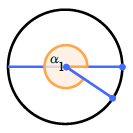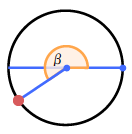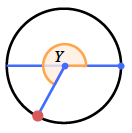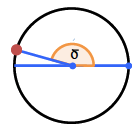### Home > PC > Chapter 5 > Lesson 5.1.2 > Problem5-24

5-24.

​​Sketch a unit circle. In your circle, sketch an angle between $0$ and $2\pi$ that has:

1. A positive cosine and a negative sine.2. A sine of $-0.5$.3. A negative cosine and a negative sine.4. A cosine of about $-0.9$ and a sine of about $0.3$.5. Could an angle have a sine equal to $0.9$ and cosine equal to $0.8$? Give an angle or explain why not.

Does $\sin^2(θ)+\cos^2(θ)=1$?